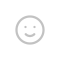## 快速傅里叶变换（FFT）

$X[k]=\sum_{n=0}^{N-1}x[n]W_N^k$

1. 周期性：$W_N^k=W_N^{k+tN}$（k,t为整数），也就是三角函数的周期性；$\sin 2\pi x=\sin 2\pi (x+k)$么。
2. 对称性：$W_N^{k+N/2}=-W_N^k$。从三角函数的角度想就是$\sin x=-\sin (x+\pi)$
3. 缩放性：$W_N^k=W_{N/2}^{k/2}$。因为代进去化简一下压根就是一回事么。

$X[k+N/2]$和$X[k]$有什么关系呢？

$X[k+N/2]=\sum_{n为偶数}^{N-2}x[n]W_N^{nk+nN/2}$。注意，n为偶数，所以$n/2$必然为整数。

$\sum_{n为偶数}^{N-2}x[n]W_N^{nk+nN/2}=\sum_{n为偶数}^{N-2}x[n]W_N^nk=G[k]$

$X[k+N/2]=\sum_{n为奇数}^{N-1}x[n]W_N^{nk+nN/2}$

$=\sum_{n为奇数}^{N-1}x[n]W_N^{nk+(n-1)N/2+N/2}$

$=\sum_{n为奇数}^{N-1}x[n]W_N^{nk+N/2}$

$=-\sum_{n为奇数}^{N-1}x[n]W_N^{nk}=-H[k]$

$X[k+N/2]=G[k+N/2]+H[k+N/2]=G[k]-H[k]$

$G[k]=\sum_{n=0}^{N/2-1}x[2n]W_N^{2kn}=\sum_{n=0}^{N/2-1}x[2n]W_{N/2}^{kn}$

$G[k]=\sum_{n=0}^{N/2-1}x[2n+1]W_N^{(2n+1)k}$

$=W_N^k\sum_{n=0}^{N/2-1}x[2n+1]W_N^{2nk}$

$=W_N^k\sum_{n=0}^{N/2-1}x[2n+1]W_{N/2}^{nk}$

## 不确定性原理

@HDTT咸鱼

（写到这里查资料的时候，发现3b1b对此也出过一期视频。那就把链接也附上吧。

 https://zhuanlan.zhihu.com/p/24318554

 https://zhuanlan.zhihu.com/p/41548628

## 发送评论编辑评论Emoji